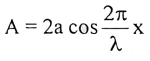# Difference between beats and interference

An interference pattern is produced when two waves of similar frequency traveling in similar direction superimpose, while beats are created when frequencies are faintly dissimilar.

Difference between beats and interference are:

Beats:

1. When two sets of waves of nearly equal frequencies and amplitude are superposed it is found that the amplitude of the resultant wave fluctuates with time from maximum to minimum. This phenomenon of fluctuation amplitude is known as beats.

2. The difference in frequencies is almost equal.

3. The phase difference of two waves with respect to time is constant.

4. The resultant amplitude with respect to time is not constant and it is,5. The resultant frequency is the average of two frequencies.

Interference:

1. When two sets of waves of the same frequency and same or nearly equal amplitude traveling through the same medium overlap, then it is found that due to superposition, they wholly or partly multiply each other at certain times and places and reinforce each other to produce a greater effect at other time and places. This phenomenon is termed interference.

2. The difference in frequencies is equal.

3. The phase difference of two waves with respect to time is not constant.

4. The resultant amplitude with respect to time is constant and it is5. The resultant frequency is equal to each of the frequencies.

In interference, the position of maximum and minimum is permanent i.e., the intensity at a given point does not change with time, but it beats the intensity of wave changes at a given point with time. For Example; when a musician needs their instrument to have the correct sound, they produce the desired frequency and try to match it with their instrument’s frequency. If the two frequencies don’t match, they interfere to produce alternating loud and soft sounds. This is how interference produces beats.